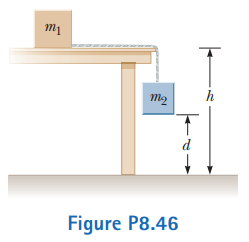# Problem: As shown in Figure P8.46, a light string that does not stretch changes from horizontal to vertical as it passes over the edge of a table. The string connects m1, a 3.50-kg block originally at rest on the horizontal table at a height h = 1.20 m above the floor, to m2, a hanging 1.90-kg block originally a distance d = 0.900 m above the floor. Neither the surface of the table nor its edge exerts a force of kinetic friction. The blocks start to move from rest. The sliding block m1 is projected horizontally after reaching the edge of the table. The hanging block m2 stops without bouncing when it strikes the floor. Consider the two blocks plus the Earth as the system.(a) Find the speed at which m1 leaves the edge of the table.(b) Find the impact speed of m1 on the floor.(c) What is the shortest length of the string so that it does not go taut while m1 is in flight?(d) Is the energy of the system when it is released from rest equal to the energy of the system just before m1 strikes the ground?(e) Why or why not?

###### FREE Expert Solution

In this problem, we'll consider the following kinematic equations:

$\overline{){\mathbf{∆}}{\mathbf{x}}{\mathbf{=}}{{\mathbf{v}}}_{{\mathbf{0}}}{\mathbf{t}}{\mathbf{+}}\frac{\mathbf{1}}{\mathbf{2}}{\mathbf{a}}{{\mathbf{t}}}^{{\mathbf{2}}}}$

88% (403 ratings)###### Problem Details

As shown in Figure P8.46, a light string that does not stretch changes from horizontal to vertical as it passes over the edge of a table. The string connects m1, a 3.50-kg block originally at rest on the horizontal table at a height h = 1.20 m above the floor, to m2, a hanging 1.90-kg block originally a distance d = 0.900 m above the floor. Neither the surface of the table nor its edge exerts a force of kinetic friction. The blocks start to move from rest. The sliding block m1 is projected horizontally after reaching the edge of the table. The hanging block m2 stops without bouncing when it strikes the floor. Consider the two blocks plus the Earth as the system.

(a) Find the speed at which m1 leaves the edge of the table.
(b) Find the impact speed of m1 on the floor.
(c) What is the shortest length of the string so that it does not go taut while m1 is in flight?
(d) Is the energy of the system when it is released from rest equal to the energy of the system just before m1 strikes the ground?
(e) Why or why not?